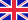•# Theories of gravitation

Autor: Source: Wikipedia

Source: Wikipedia. Pages: 117. Chapters: Theory of everything, Kaluza-Klein theory, Quantum gravity, Cosmological constant, Le Sage's theory of gravitation, Alternatives to general relativity, Introduction to general relativity, An Exceptionally Simple Theory... Viac o knihe

Na objednávku, dodanie 2-4 týždne

23.97 €

bežná cena: 28.20 €

## O knihe

Source: Wikipedia. Pages: 117. Chapters: Theory of everything, Kaluza-Klein theory, Quantum gravity, Cosmological constant, Le Sage's theory of gravitation, Alternatives to general relativity, Introduction to general relativity, An Exceptionally Simple Theory of Everything, Einstein-Cartan theory, Newton's law of universal gravitation, Nordström's theory of gravitation, Self-creation cosmology, Modified Newtonian dynamics, Mechanical explanations of gravitation, Supergravity, Large extra dimension, Parameterized post-Newtonian formalism, Stochastic electrodynamics, Mach's principle, History of gravitational theory, Gauss' law for gravity, F(R) gravity, Brans-Dicke theory, Polarizable vacuum, Dark fluid, Unified field theory, Tensor-vector-scalar gravity, Twistor theory, Scalar-tensor-vector gravity, Gravitational instanton, Geometrodynamics, Scalar theories of gravitation, Einstein aether theory, Entropic gravity, Gravitational field, Classical theories of gravitation, Bimetric theory, Lovelock theory of gravity, Teleparallelism, Yilmaz theory of gravitation, Chronology protection conjecture, Nonsymmetric gravitational theory, Newton-Cartan theory, Conformal gravity, Semiclassical gravity, DGP model, Massive gravity, Induced gravity, Whitehead's theory of gravitation, Higher-dimensional Einstein gravity, AQUAL, Mach principle, Horava-Lifshitz gravity, Hoyle-Narlikar theory of gravity, Universal extra dimension, Gauss-Bonnet gravity, Graviscalar, Democratic principle, Plebanski action, Bi-scalar tensor vector gravity, Composite gravity, Nuts and bolts. Excerpt: Le Sage's theory of gravitation is a kinetic theory of gravity originally proposed by Nicolas Fatio de Duillier in 1690 and later by Georges-Louis Le Sage in 1748. The theory proposed a mechanical explanation for Newton's gravitational force in terms of streams of tiny unseen particles (which Le Sage called ultra-mundane corpuscles) impacting all material objects from all directions. According to this model, any two material bodies partially shield each other from the impinging corpuscles, resulting in a net imbalance in the pressure exerted by the impact of corpuscles on the bodies, tending to drive the bodies together. This mechanical explanation for gravity never gained widespread acceptance, although it continued to be studied occasionally by physicists until the beginning of the 20th century, by which time it was generally considered to be conclusively discredited. The theory posits that the force of gravity is the result of tiny particles (corpuscles) moving at high speed in all directions, throughout the universe. The intensity of the flux of particles is assumed to be the same in all directions, so an isolated object A is struck equally from all sides, resulting in only an inward-directed pressure but no net directional force (P1). With a second object B present, however, a fraction of the particles that would otherwise have struck A from the direction of B is intercepted, so B works as a shield, i.e. from the direction of B, A will be struck by fewer particles than from the opposite direction. Likewise B will be struck by fewer particles from the direction of A than from the opposite direction. One can say that A and B are "shadowing" each other, and the two bodies are pushed toward each other by the resulting imbalance of forces (P2). Thus the apparent attraction between bodies is, according to this theory, actually a diminished push from the direction of other bodies, s...

• Vydavateľstvo: Books LLC, Reference Series
• Rok vydania: 2011
• Formát: Paperback
• Rozmer: 246 x 189 mm
• Jazyk: Anglický jazyk
• ISBN: 9781157644613

Generuje redakčný systém BUXUS CMS spoločnosti ui42.